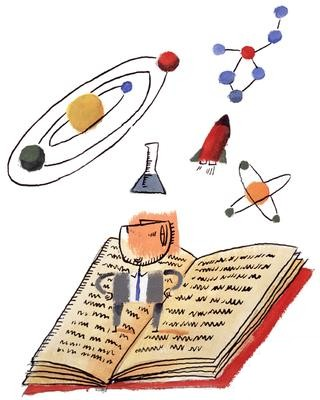eScience courses (M3)

### M3 Physics - ว.23204 Unit 3 - Forces & MotionUnit 3 - Forces & Motion
Covers the topics:

• Newton's first law - Inertia
• Newton's second law - F=mxa
• Newton's third law of motion - Action-Reaction
• Buoyant force in liquids
• Types of friction (Static, Kinetic, Rolling, Fluid)
• Moment of force
• Types pf Motion